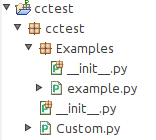# Error when running custom model in notebook

Hi,

I’ve come across a strange error when running a custom model in the notebook. The code is the same as this in a previously raised issue (with the correction to address that issue), shown below (needs to be in packages organised as before)…Custom.py:

``````from bokeh.plotting import Figure
from bokeh.layouts import Column

class Custom(Column):

__view_model__ = 'Column'
__subtype__ = 'Custom'
__view_module__ = '__main__'

def __init__(self):

super().__init__()

plot = Figure(plot_height=500, plot_width=500)
plot.circle([1, 2, 3], [3, 2, 1], size=20)

self.children.append(plot)
``````

example.py:

``````from bokeh.plotting import show
from cctest.Custom import Custom

custom = Custom()
show(custom)
``````

(The init.py under both cctest and Examples is empty.)

example.py runs successfully at the command line. However if I run the following code in the notebook:

``````from bokeh.plotting import show
from bokeh.io import output_notebook
from cctest.Custom import Custom
output_notebook()
custom = Custom()
show(custom)
``````

I get the error:
AttributeError: module ‘main’ has no attribute ‘file

Note that if I don’t have a heirarchy, i.e. enter the following in the notebook:

``````from bokeh.plotting import Figure, show
from bokeh.layouts import Column
from bokeh.io import output_notebook
output_notebook()

class Custom(Column):

__view_model__ = 'Column'
__subtype__ = 'Custom'
__view_module__ = '__main__'

def __init__(self):

super().__init__()

plot = Figure(plot_height=500, plot_width=500)
plot.circle([1, 2, 3], [3, 2, 1], size=20)

self.children.append(plot)

custom = Custom()
show(custom)
``````

then it works fine.

Any help appreciated!

Thanks,
Marcus.

@Marcus_Donnelly For this, I would suggest comment on the issue directly.

OK, I’ve just done that @Bryan. I can’t seem to delete this new issue but happy for someone else to…

@Marcus_Donnelly Sorry, to be clear I meant the GitHub Issue

Ah, sorry, I misunderstood, will do that now…